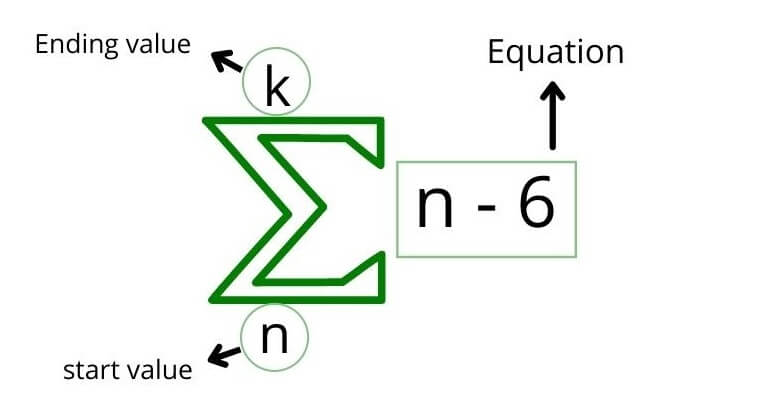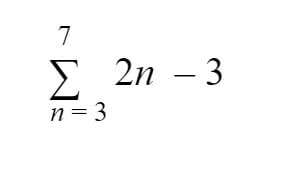# Summation Calculator

To find the sum of a series, fill the respected boxes with starting and ending values, and equation in the summation notation or sigma calculator.

This will be calculated:## Summation Calculator

This Summation calculator (solver) computes the partial sum of a series. Users can choose between

• Simple sum calculator (Enter numbers simply)
• Sigma notation calculator (equation needed)

## What is Summation?

Summation (aka sum) is the result obtained after adding the numbers of a sequence.

Summation notation (sigma notation) is used to represent a long sum in a single expression.## Summation notation Example:

Solve:Solution:

Step 1: Understand the operations:

Values = 3 to 7 = 3, 4, 5, 6, 7

Equation = 2n - 3

Step 2: Put the values in the equation:

For n = 3

2(3) - 3 = 3

For n = 4

2(4) - 3 = 5

For n = 5

2(5) - 3 = 7

For n = 6

2(6) - 3 = 9

For n = 7

2(7) - 3 = 11

Step 3: Add all the results:

= 3 + 5 + 7 + 9 + 11

= 35